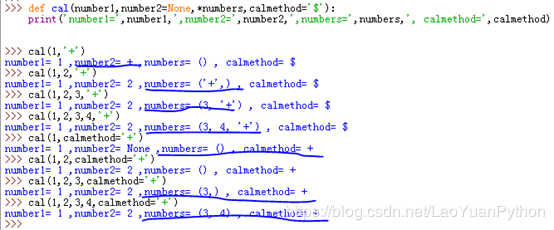Jquery中文网 www.jquerycn.cn
Jquery中文网 >  Python编程  >  Python入门  >  正文 详解Python函数中参数带星号是什么意思

# 详解Python函数中参数带星号是什么意思

jquery中文网为您提供详解Python函数中参数带星号是什么意思等资源，欢迎您收藏本站，我们将为您提供最新的详解Python函数中参数带星号是什么意思资源Python的函数支持可变不定数量的参数模式，这种将不定数量实参在调用时传递给函数，函数将其识别并保存到指定形参的过程称为参数收集：

1、带一个星号的参数收集模式

```\>>> def cal(number1,number2=None,*numbers,calmethod='\$'):

print('number1=',number1,',number2=',number2,',numbers=',numbers,', calmethod=',calmethod)```

```\>>> cal(1,' ')

number1= 1 ,number2=   ,numbers= () , calmethod= \$

\>>> cal(1,2,' ')

number1= 1 ,number2= 2 ,numbers= (' ',) , calmethod= \$

\>>> cal(1,2,3,' ')

number1= 1 ,number2= 2 ,numbers= (3, ' ') , calmethod= \$

\>>> cal(1,2,3,4,' ')

number1= 1 ,number2= 2 ,numbers= (3, 4, ' ') , calmethod= \$

\>>> cal(1,calmethod=' ')

number1= 1 ,number2= None ,numbers= () , calmethod=

\>>> cal(1,2,calmethod=' ')

number1= 1 ,number2= 2 ,numbers= () , calmethod=

\>>> cal(1,2,3,calmethod=' ')

number1= 1 ,number2= 2 ,numbers= (3,) , calmethod=

\>>> cal(1,2,3,4,calmethod=' ')

number1= 1 ,number2= 2 ,numbers= (3, 4) , calmethod=

\>>>```2、带两个星号的参数收集模式

```\>>> def cal(calmethod=' ',*topnopers,**lastnopers):

print('运算符=',calmethod,',前几个运算参数为=',topnopers,',最后几个运算参数=',lastnopers)

\>>> cal(' ',1,2,3,4,n5=5,n6=6,n7=7)```

[]